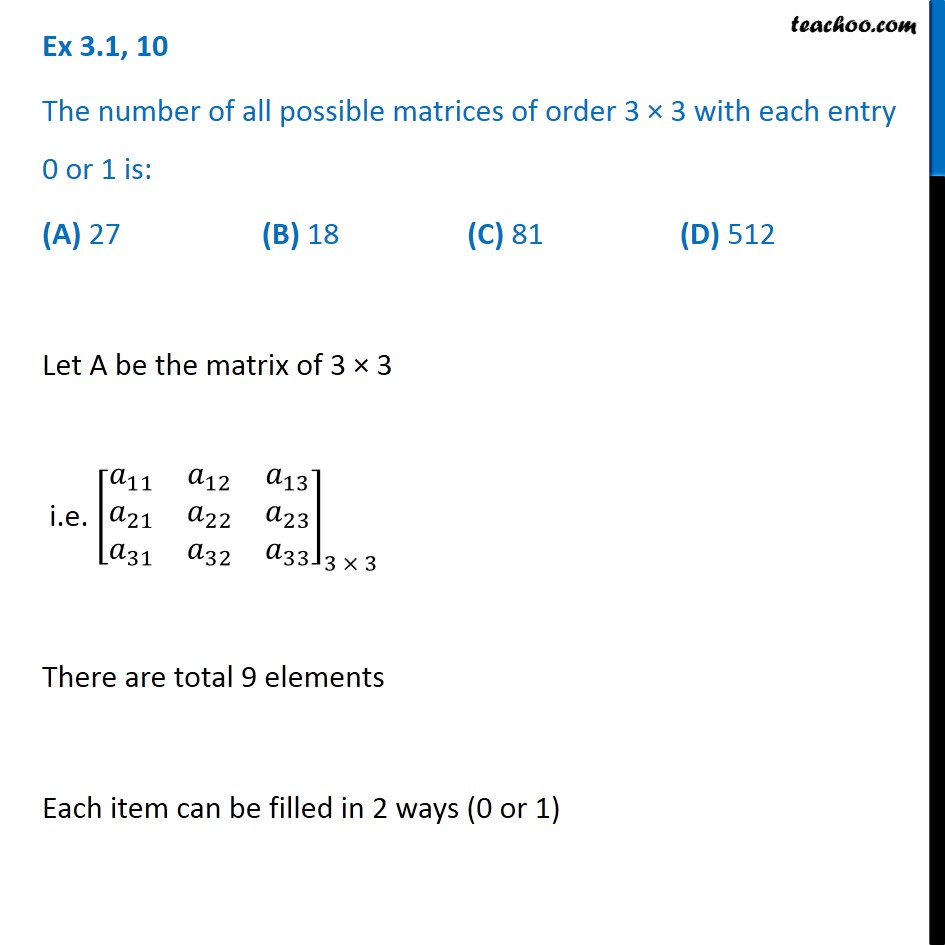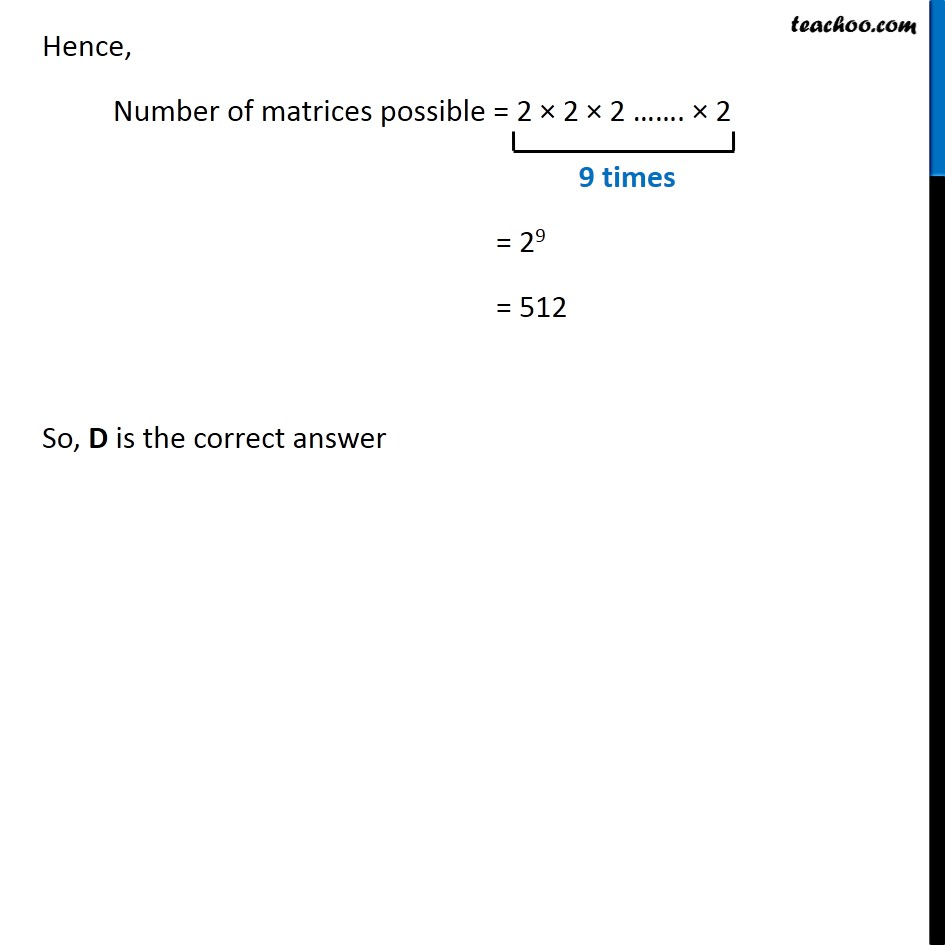1. Chapter 3 Class 12 Matrices (Term 1)
2. Concept wise
3. Formation and order of matrix

Transcript

Ex 3.1, 10 The number of all possible matrices of order 3 × 3 with each entry 0 or 1 is: (A) 27 (B) 18 (C) 81 (D) 512 Let A be the matrix of 3 × 3 i.e. [■8(𝑎_11&𝑎_12&𝑎_13@𝑎_21&𝑎_22&𝑎_23@𝑎_31&𝑎_32&𝑎_33 )]_(3 × 3) There are total 9 elements Each item can be filled in 2 ways (0 or 1) Hence, Number of matrices possible = 2 × 2 × 2 ……. × 2 = 29 = 512 So, D is the correct answer